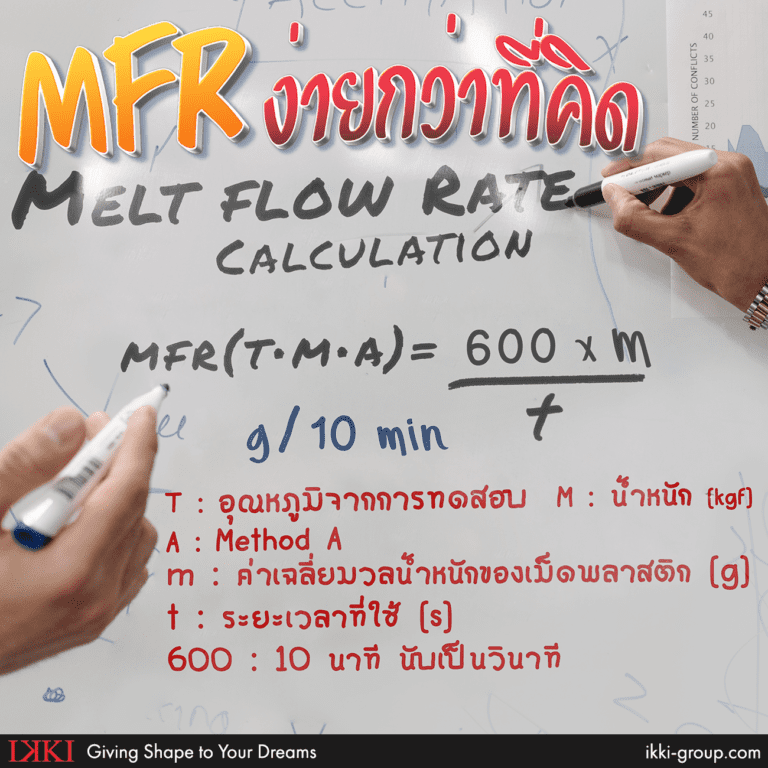# What is MFR and how to calculate MFR

## Before we get into the calculation we need to understand first…

Melt Flow Rate is the flow rate of plastic pallets (Thermoplastic Resin) in g/10 min. That means the total mass of plastic pallets (gram) that flow in 10 mins through the Capillary. The capillary is being controlled by size of diameter, length of total weight is being pressed by the standard and controlled temperature.

## Why do you need to calculate MFR before using...

Each type of plastic pallets or polymers material may have differences in properties. For industrial work, it is quite common to face the problem during injection molding due to the reason of deviation on information that provided from plastic pallet seller (Example of common problem in injection molding)

Frankly speaking of the MFR or Melt flow rate, if we are about to simplify our explanation it would be just… Plastic pallets that are melted by controlled standard temperature and being pressed by weight of Piston. (Standard weight is 2.6 kg, 5kg, etc.) And from testing the melt flow rate user will be able to directly  tell the properties of plastic before using

Instrument to be used in the MFR: Melt flow testing

Calculation of MFR Melt Flow Rate: Method A or Procedure A

Method A that was meant to be Manual Calculation methodMFR is consisted of: T = testing temperature (C), M = mass (kgf),  A = Method A
And A = Method A and Method A is equal to : m = average of total mass of plastic pallet (g), t = time used (s), 600 = total second of 10 mins

Standard testing table for Thermoplastic Resin that can be used together with Melt Flow Tester